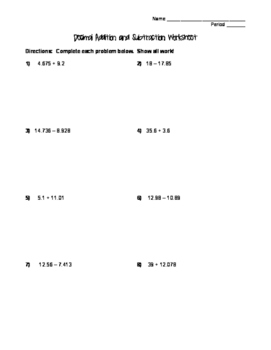# Decimal Addition & Subtraction Practice Worksheet - Positive Numbers OnlySubject
Resource Type
File Type

PDF

(86 KB|1 page)
Standards
• Product Description
• StandardsNEW
This is a worksheet with 8 problems that covers decimal addition and subtraction. You could use this as a homework page, a quiz, in-class work, or as a station activity!

This worksheet contains 4 decimal addition problems and 4 decimal subtraction problems. All problems and answers are positive numbers only.

Additionally, the first page of this document has the problems only. The second page is the same as the first page but also has small grids under each problem. Providing a grid can really help students learn to line up decimal points accurately! This would be a great way to differentiate within your classroom.
Apply and extend previous understandings of addition and subtraction to add and subtract rational numbers; represent addition and subtraction on a horizontal or vertical number line diagram.
Fluently add, subtract, multiply, and divide multi-digit decimals using the standard algorithm for each operation.
Add, subtract, multiply, and divide decimals to hundredths, using concrete models or drawings and strategies based on place value, properties of operations, and/or the relationship between addition and subtraction; relate the strategy to a written method and explain the reasoning used.
Total Pages
1 page
Not Included
Teaching Duration
N/A
Report this Resource to TpT
Reported resources will be reviewed by our team. Report this resource to let us know if this resource violates TpT’s content guidelines.